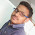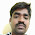MVC ASP.NET C# SQL Server WCF Written Test HR Round Subscribe C# Videos C# Programs Buy DVD

### C# program to print even numbers

This is a sample C# program to print even numbers. There are 2 ways to to print even numbers.
1. Start at Zero. Increment the value by 2, print it. Do this until you reach your target number.
2. Start at Zero. Divide the number by 2. If the remainder is zero, then you know the number is even. So print it and then increment it by 1 and divide the number by 2 again. Repeat this until we reach our target number.

This program can also be used to print odd numbers by making a minor logic change. Initialize the targetNumber variable to 1 instead of 0. If you are using dividing logic, then print the number if the remainder is not zero.

Note: This program will crash and throws an exception, if you enter a very big number or a string. To handle exceptions, we can make use of try catch blocks. We will look at this in another program.

using System;
namespace SamplePrograms
{
class EvenNumbers
{
public static void Main()
{
// Prompt the user to enter a target number. Target number is the
// number untill which the user want to have even and odd numbers printed

Console.WriteLine("Please enter your target");

// Declare a variable to hold the target number
int targetNumber = 0;

// Retrieve, Convert and store the target number
targetNumber = Convert.ToInt32(Console.ReadLine());

// Use a FOR or WHILE loop to print the even numbers, until our target number
for (int i = 0; i <= targetNumber; i = i + 2)
{
Console.WriteLine(i);
}

// You can also check if a number is even, by dividing it by 2.
//for (int i = 0; i <= targetNumber; i++)
//{
//    if ((i % 2) == 0)
//    {
//        Console.WriteLine(i);
//    }
//}

// You can also use a while loop to do the same as shown below.
//int start = 0;
//while (start <= targetNumber)
//{
//    Console.WriteLine(start);
//    start = start + 2;
//}
// This line is to make the program wait for user input, instead of immediately closing
Console.ReadLine();

}
}
}

#### 6 comments:

1.thank you so much sir

2.This comment has been removed by the author.

3.One more and possibly the most elegant way to determine even/odd numbers is by using the C# bitwise & (and) operator instead of dividing by 2, like so:

if ((i & 1) == 1)
{
Console.WriteLine(i); // odd number
}
// or
if ((i & 1) == 0)
{
Console.WriteLine(i); // even number
}

This works because odd numbers always have 1 in the loweset bit of their binary representation, whereas even numbers always have 0.

1 is binary 0...00000001, so the bitwise & Operator will zero out all bits it reads from i (x & 0 == 0) except for the lowest one:

– For odd i, its lowest bit (1) will also lead to 1 in the result's lowest bit (1 & 1 == 1), so the result will be 0...00000001 == 1.

– For even i, its lowest bit (0) will also lead to 0 in the result's lowest bit (0 & 1 == 0), so the result will be 0...00000000 == 0.

Don P

4.superbbbbbbbbbb

5.6.